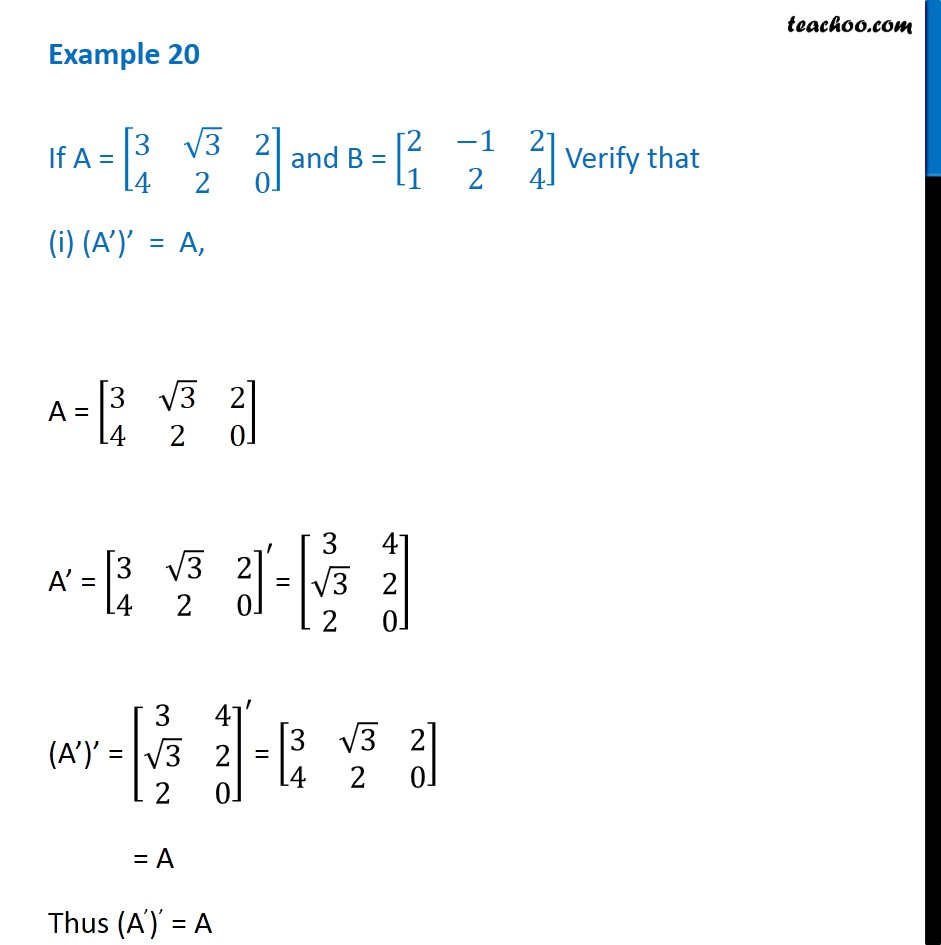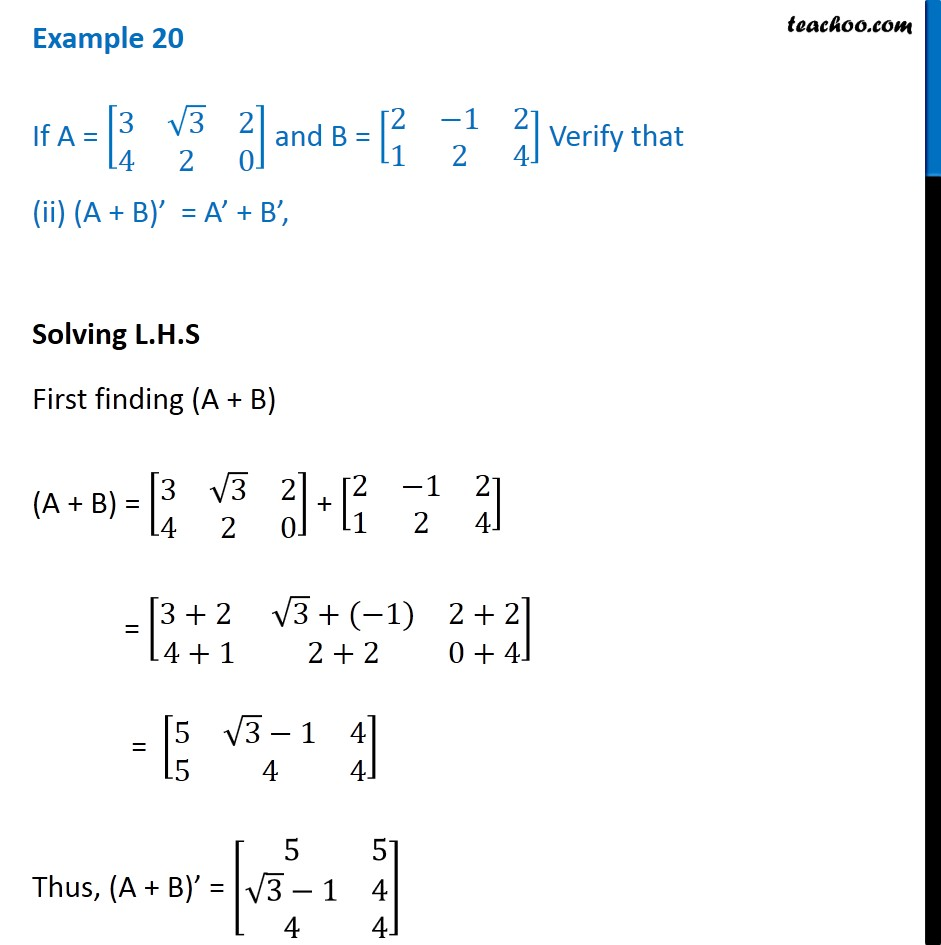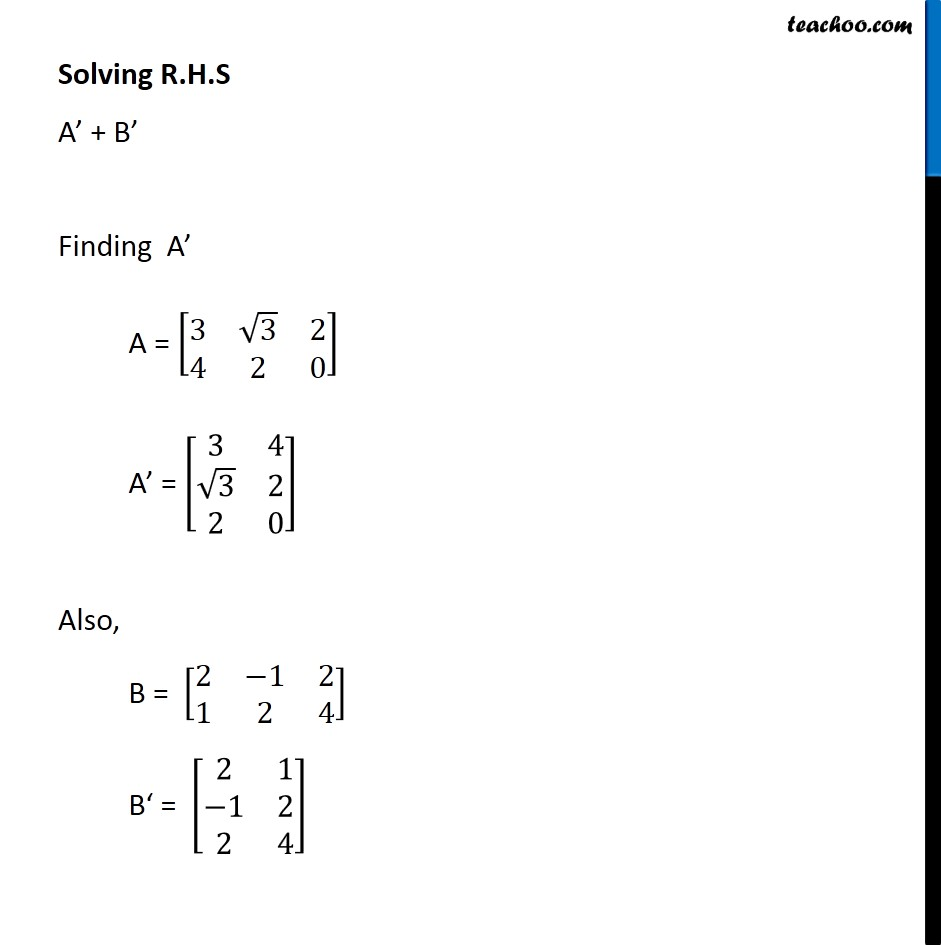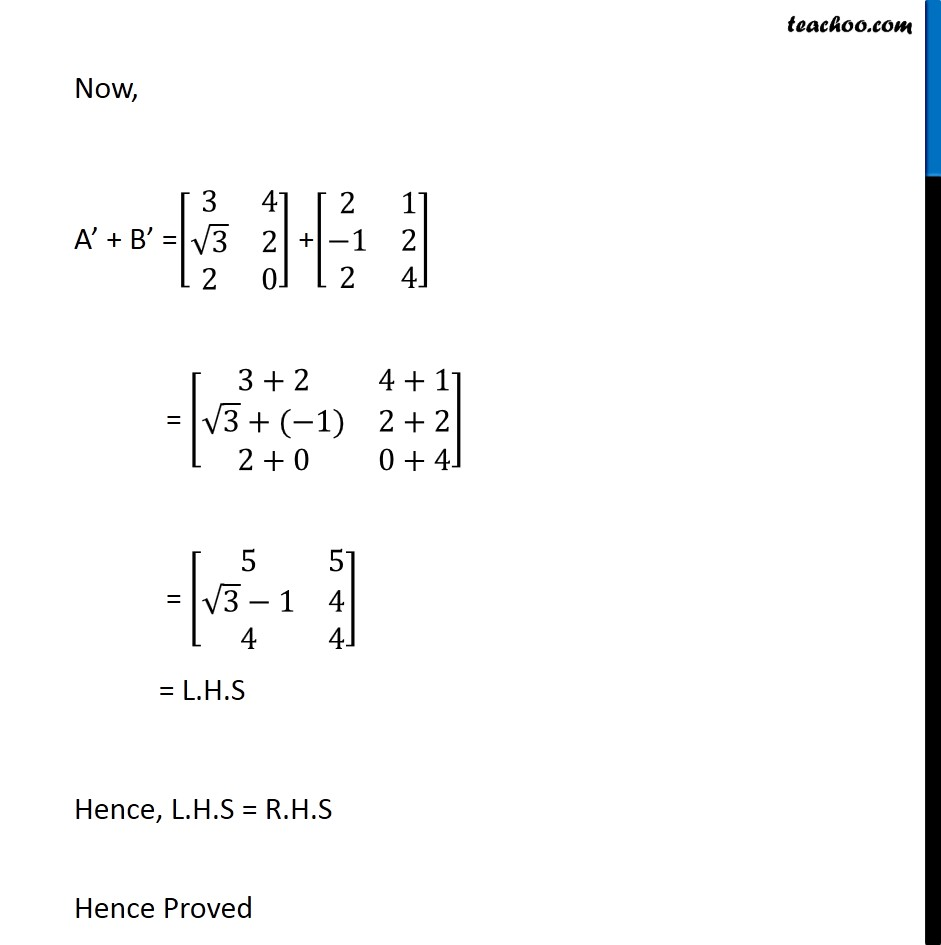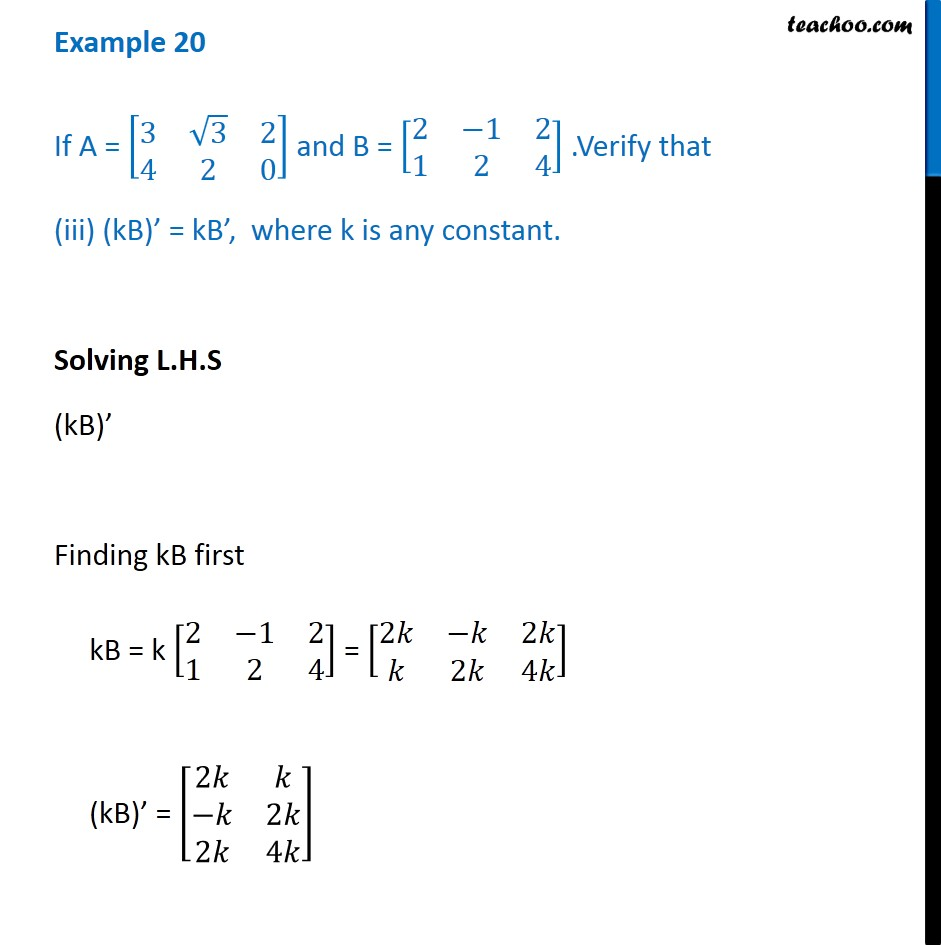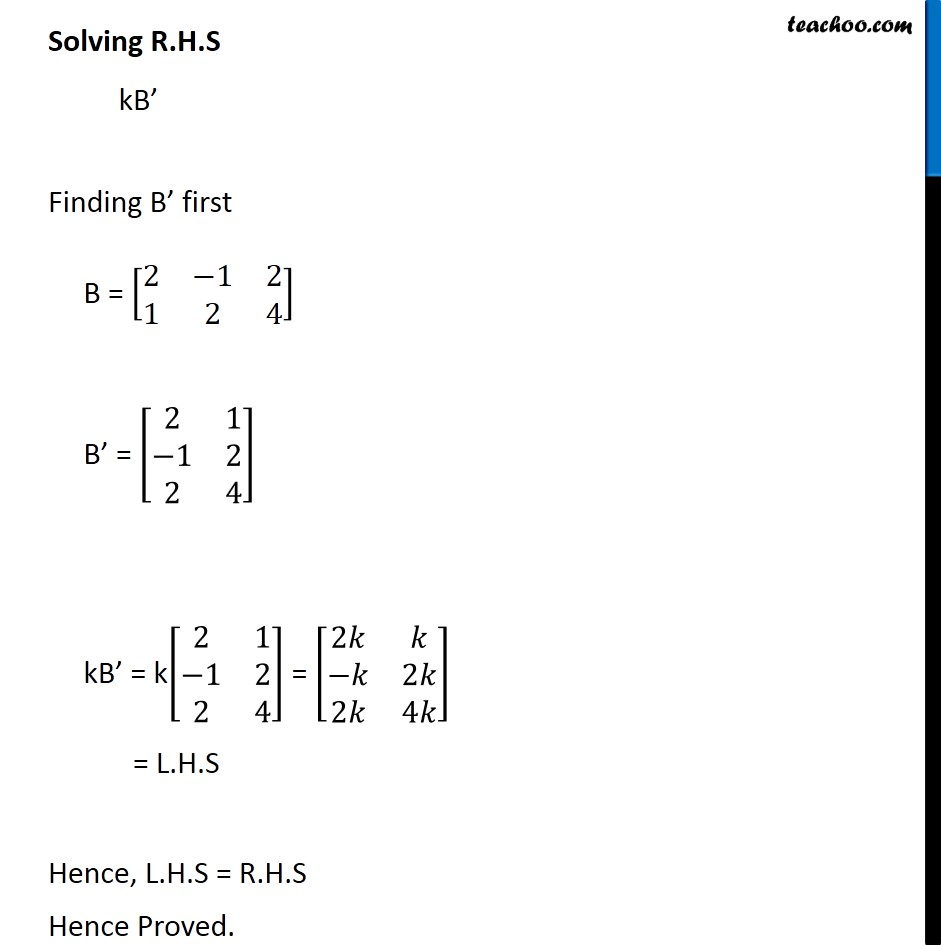1. Chapter 3 Class 12 Matrices (Term 1)
2. Serial order wise
3. Examples

Transcript

Example 20 If A = [■8(3&√3&2@4&2&0)] and B = [■8(2&−1&2@1&2&4)] Verify that (i) (A’)’ = A, A = [■8(3&√3&2@4&2&0)] A’ = [■8(3&√3&2@4&2&0)]^′= [■8(3&4@√3&2@2&0)] (A’)’ = [■8(3&4@√3&2@2&0)]^′= [■8(3&√3&2@4&2&0)] = A Thus (A’)’ = A Example 20 If A = [■8(3&√3&2@4&2&0)] and B = [■8(2&−1&2@1&2&4)] Verify that (i) (A’)’ = A, A = [■8(3&√3&2@4&2&0)] A’ = [■8(3&√3&2@4&2&0)]^′= [■8(3&4@√3&2@2&0)] (A’)’ = [■8(3&4@√3&2@2&0)]^′= [■8(3&√3&2@4&2&0)] = A Thus (A’)’ = A Example 20 If A = [■8(3&√3&2@4&2&0)] and B = [■8(2&−1&2@1&2&4)] Verify that (ii) (A + B)’ = A’ + B’, Solving L.H.S First finding (A + B) (A + B) = [■8(3&√3&2@4&2&0)] + [■8(2&−1&2@1&2&4)] = [■8(3+2 &√3+(−1)&2+2@4+1&2+2&0+4)] = [■8(5&√3−1&4@5&4&4)] Thus, (A + B)’ = [■8(5&5@√3−1&4@4&4)] Solving R.H.S A’ + B’ Finding A’ A = [■8(3&√3&2@4&2&0)] A’ = [■8(3&4@√3&2@2&0)] Also, B = [■8(2&−1&2@1&2&4)] B‘ = [■8(2&1@−1&2@2&4)] Now, A’ + B’ =[■8(3&4@√3&2@2&0)] +[■8(2&1@−1&2@2&4)] = [■8(3+2&4+1@√3+(−1)&2+2@2+0&0+4)] = [■8(5&5@√3−1&4@4&4)] = L.H.S Hence, L.H.S = R.H.S Hence Proved Example 20 If A = [■8(3&√3&2@4&2&0)] and B = [■8(2&−1&2@1&2&4)] .Verify that (iii) (kB)’ = kB’, where k is any constant. Solving L.H.S (kB)’ Finding kB first kB = k [■8(2&−1&2@1&2&4)] = [■8(2𝑘&−𝑘&2𝑘@𝑘&2𝑘&4𝑘)] (kB)’ = [■8(2𝑘&𝑘@−𝑘&2𝑘@2𝑘&4𝑘)] Solving R.H.S kB’ Finding B’ first B = [■8(2&−1&2@1&2&4)] B’ = [■8(2&1@−1&2@2&4)] kB’ = k[■8(2&1@−1&2@2&4)] = [■8(2𝑘&𝑘@−𝑘&2𝑘@2𝑘&4𝑘)] = L.H.S Hence, L.H.S = R.H.S Hence Proved.

Examples

Chapter 3 Class 12 Matrices (Term 1)
Serial order wise